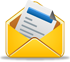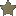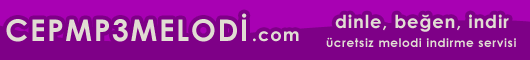Hoşgeldiniz, bugün 31 May 2020
aktif üye 8,903
SEN DE YAZ
HazırKod ziyaretçilerinin katkıları bizim için çok önemli!Sizde hazırkod üyesi olun, ekleyeceğiniz kodlar yapacağınız yorumlarla hazirkod.com'a katkıda bulunun.
ETİKETLER

# Programalama > JAVA SCRIPT

Etiketler: buton, ?eklinde, saatOrt. 0
Puan ver:
```<SCRIPT LANGUAGE="JavaScript">

<!-- Begin
day = new Date();
miVisit = day.getTime();
function clock() {
dayTwo = new Date();
hrNow = dayTwo.getHours();
mnNow = dayTwo.getMinutes();
scNow = dayTwo.getSeconds();
miNow = dayTwo.getTime();
if (hrNow == 0) {
hour = 12;
ap = " AM";
} else if(hrNow <= 11) {
ap = " AM";
hour = hrNow;
} else if(hrNow == 12) {
ap = " PM";
hour = 12;
} else if (hrNow >= 13) {
hour = (hrNow - 12);
ap = " PM";
}
if (hrNow >= 13) {
hour = hrNow - 12;
}
if (mnNow <= 9) {
min = "0" + mnNow;
}
else (min = mnNow)
if (scNow <= 9) {
secs = "0" + scNow;
} else {
secs = scNow;
}
time = hour + ":" + min + ":" + secs + ap;
document.form.button.value = time;
self.status = time;
setTimeout('clock()', 1000);
}
function timeInfo() {
milliSince = miNow;
milliNow = miNow - miVisit;
secsVisit = Math.round(milliNow / 1000);
minsVisit = Math.round((milliNow / 1000) / 60);
alert("There have been " + milliSince + " milliseconds since midnight, January 1, 1970. "
+ "You have spent " + milliNow + " of those milliseconds on this page. "
+ ".... About " + minsVisit + " minutes, and "
+ secsVisit + " seconds.");
}
document.write("<form name=\"form\">"
+ "<input type=button value=\"Click for info!\""
+ " name=button onClick=\"timeInfo()\"></form>");
onError = null;
clock();
// End -->
</SCRIPT>```Yorumlar                 Yorum Yaz
Bu hazır kod'a ilk yorumu siz yapın!
KATEGORİLER
ASP - 240
ASP.NET - 24
C# - 75
C++ - 174
CGI - 8
DELPHI - 247
FLASH - 49
HTML - 536
PASCAL - 246
PERL - 11
PHP - 160
WML - 9
XML - 2